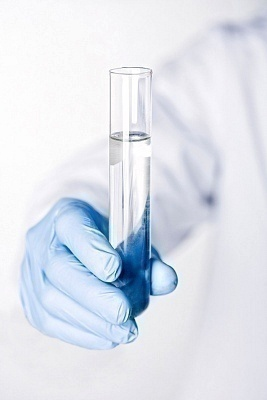•• # Molality

Molality is the number of moles in a solute per kilogram of solvent. It is often confused with the term molarity, which is an alternative unit for concentration that measures the number of moles of solute per liter of solution. Molality is commonly used when experiments require significant temperature changes. This is because a solute’s molality remains the same with increasing temperature, since it is based on mass instead of volume, unlike molarity.

## How to Calculate Molality

Step 1 – Divide the solute mass by the solute’s molecular weight. E.g. For Br2, if 10 grams of the substance are dissolved in 1L of cyclohexane, multiply the molecular weight of Br by 2, then divide the mass of Br2 in the solution by the molecular weight. The answer is 0.063 moles of Br2.Step 2 – Multiply the solvent’s volume and density to calculate its overall mass. In this case, the cyclohexane density is 0.779 KG/L at room temperature. Multiply this number by the volume in order to determine the solvent’s mass.

Step 3 – Divide the total moles of the solute by the mass of the solution given in kilograms to determine the solute’s molality. In this example, 0.063 moles of Br2 is divided by 0.779 kg of cyclohexane to arrive at a 0.080 m molality of the solute.

## How to Calculate Molality Given Molarity

Chemistry often requires a solute’s molality to be calculated when given the molarity.

Step 1 – Determine the density of the solute in the substance whose molality will be determined, given the molarity. Fill a graduated cylinder to the 5 ml mark with the solute and measure its mass. Subtract the empty cylinder’s weight to determine the solute’s mass.

Step 3 – Determine the solute’s molecular mass by adding up the atoms’ atomic masses as found on the Periodic Table of Elements.

Step 4 – Determine the number of grams of solute in one liter of the solution by multiplying its molarity by the substance’s molecular weight.

Step 5 – Determine the solute’s volume in a liter by dividing the grams in one liter of the mixture by the solute’s density.

Step 6 – Calculate the volume of water per liter of the solution by subtracting the volume of the solute from 1000 ml.

Step 7 – Divide the total number of moles of the solute by the kilograms to calculate the molality.

1. #### Irene

16 March, 2011 at 5:47 pm

Your intro text reads, “Many times it is confused with the term molarity which is an alternative unit for concentration measuring the number of mols of solute per liter of solvent”.   Please correct that – it should make it clear that molarity is the number of mols of solute per liter of SOLUTION.  See page 412 of Modern Chemistry by Holt, Rinehart, Winston or p. 227 of Chemistry in Context, 5th ed. from the American Chemical Society, or any number of other sources.
Thanks.